# Section 11 Improving the Model Fit

When we developed the theory for linear models, we used the following assumptions:

• Linear relationship between inputs and outputs: $$y \approx x^\top \beta$$

• Independence of errors: the $$\varepsilon_i = y_i - (X\beta)_i$$ are independent of each other.

• Normally distributed errors: $$\varepsilon_i \sim \mathcal{N}(0, \sigma^2)$$ for all $$i$$. In particular, the variance of the $$\varepsilon_i$$ does not depend on $$i$$.

The diagnostic plots mentioned in section 8.1 can often reveal if the data do not fit these modelling assumptions. In cases where the assumptions are violated, sometimes a linear model can still be used if the data is transformed first.

## 11.1 Linearising the Mean

If the model mean is a non-linear function of the inputs, we can sometimes transform the variables to achieve a linear relationship. We list some examples of non-linear models which can be transformed to linear models:

nonlinear model transformation linear Model
$$y \approx \beta_0 x^{\beta_1}$$ $$x'=\log(x)$$, $$y'=\log(y)$$ $$y' \approx \log(\beta_0) + \beta_1 x'$$
$$y \approx \beta_0 e^{\beta_1 x}$$ $$y'=\log y$$ $$y' \approx \log \beta_0 +\beta_1 x$$
$$y \approx \beta_0+\beta_1\log x$$ $$x'=\log x$$ $$y \approx \beta_0+\beta_1 x'$$
$$y \approx \frac{x}{\beta_0 x-\beta_1}$$ $$x'=1/x$$, $$y'=1/y$$ $$y' \approx \beta_0-\beta_1 x'$$

In all such cases we also would need to check the residuals of the transformed models, to see whether linear regression can be used for the transformed model.

## 11.2 Stabilising the Variance

The assumption of constant variance is a basic requirement of regression analysis. A common reason for the violation of this assumption is for the response variable $$y$$ to follow a distribution in which the variance depends on $$y$$ or $$\mathbb{E}(y)$$ and thus on $$x$$.

Example 11.1 The error in our model $\begin{equation*} Y = \beta_0 + \beta_1 x_1 + \beta_2 x_2 + \cdots + \beta_p x_p + \varepsilon = x^\top \beta + \varepsilon \end{equation*}$ is sometimes called additive error, since it is added to the model mean $$x^\top\beta$$. Sometimes the error is instead given in percentages of the quantity of interest. In these cases we speak of multiplicative error. This can, for example, be modelled as $\begin{equation*} Y = \bigl(\beta_0 + \beta_1 x_1 + \beta_2 x_2 + \cdots + \beta_p x_p\bigr) \exp(\varepsilon) = x^\top \beta \exp(\varepsilon). \end{equation*}$ For $$\varepsilon= 0$$ we have $$\exp(0) = 1$$ and thus $$Y = x^\top \beta$$. Similarly, for small $$\varepsilon$$ we have $$Y \approx x^\top \beta$$, but the variance is now proportional to $$(x^\top\beta)^2$$ instead of being constant. Also, since the exponential is nonlinear we only have $$\mathbb{E}(Y) \approx x^\top\beta$$ instead of strict equality.

Some commonly-used variance stabilising transformations are:

variance transformation
$$\sigma^2 = \text{constant}$$ no transformation
$$\sigma^2 \propto y$$ $$y' = \sqrt{y}$$
$$\sigma^2 \propto y^2$$ $$y' = \log y$$
$$\sigma^2 \propto y^3$$ $$y' = \frac{1}{\sqrt{y}}$$
$$\sigma^2 \propto y^4$$ $$y' = \frac{1}{y}$$
$$\sigma^2 \propto y(1-y)$$ where $$y \in [0,1]$$ $$y' = \arcsin(\sqrt{y})$$

Of course we do not have accurate knowledge of the relationship, but it can be diagnosed from the residual plots and transformations can be selected by experimenting with different choices. Any of these transformations will also affect the mean and we need to check the model fit for the transformed data, to see whether the transformed data can still reasonably be described by a linear model.

Example 11.2 The last transformation in the table above corresponds to the case of binomial sampling: If $$x = p$$ and $$Y \sim B(n, p) / n$$ then we have $$\mathbb{E}(Y) = n p / n = x$$ and a linear model may be appropriate. But we also have $$\mathop{\mathrm{Var}}(Y) = p (1 - p) / n \propto \mathbb{E}(Y) \bigl( 1- \mathbb{E}(Y) \bigr)$$, so the assumption of constant variance is violated.

We try to apply the transformation suggested in the table. The function $$\arcsin$$ is the inverse of the sine function. In R this function is available as asin(). To get some intuition about this transformation, we plot the function $$y \mapsto \arcsin(\sqrt{y})$$:

y <- seq(0, 1, l=101)
plot(y, asin(sqrt(y)), type = "l",
ylab = expression(y * minute))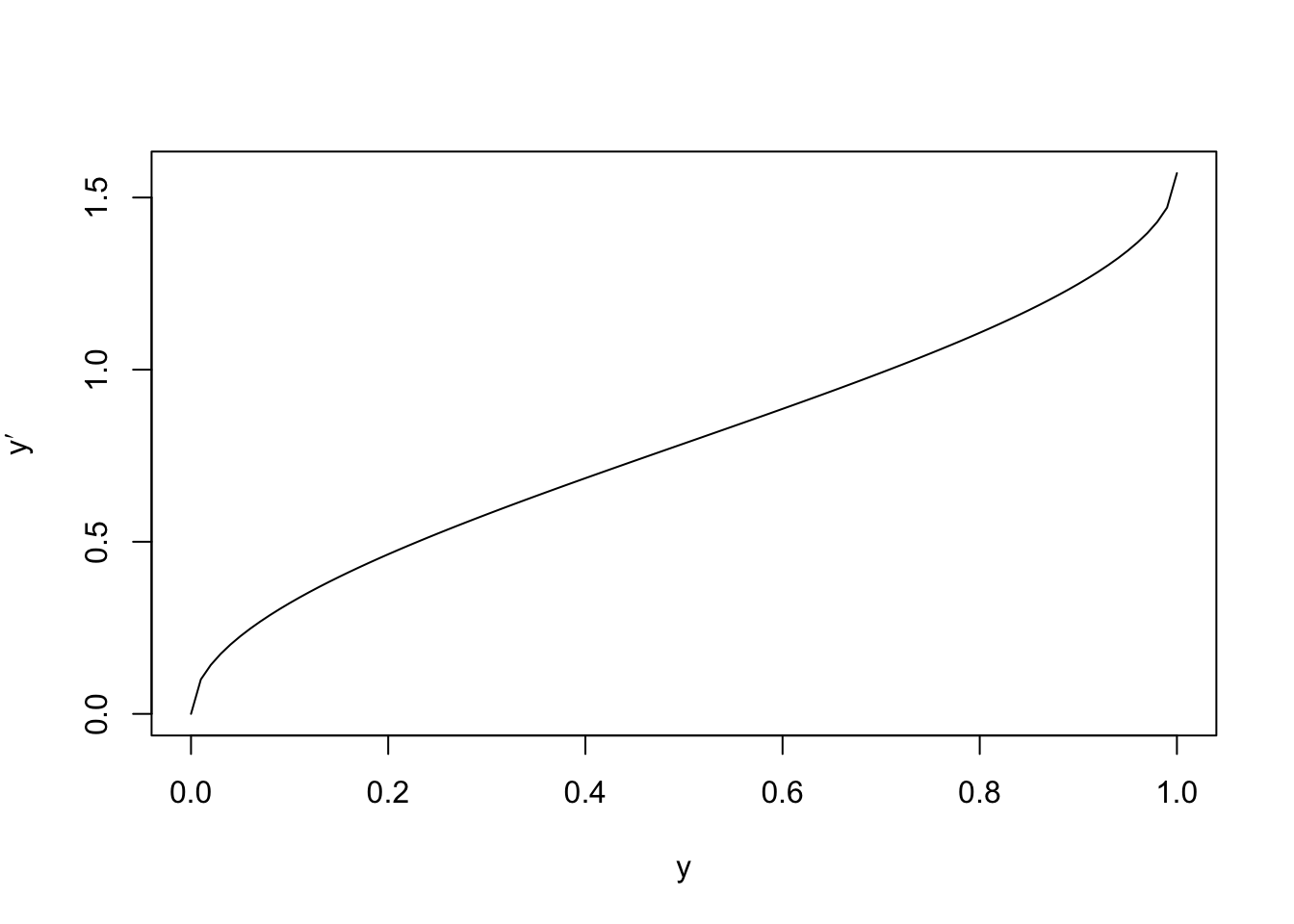We can see that the transformation is approximately linear for most of the interval, but has a steeper slope near the edges. The effect of this is to increase the size of fluctations for small and large $$y$$-values. We now consider residual plots for the original and transformed data, for a simulated dataset:

n <- 500
x <- runif(n, 0, 1)
y <- rbinom(n, 100, x) / 100

par(mfrow = c(1, 2))

m1 <- lm(y ~ x)
plot(fitted(m1), resid(m1))

y.prime <- asin(sqrt(y))
m2 <- lm(y.prime ~ x)
plot(fitted(m2), resid(m2))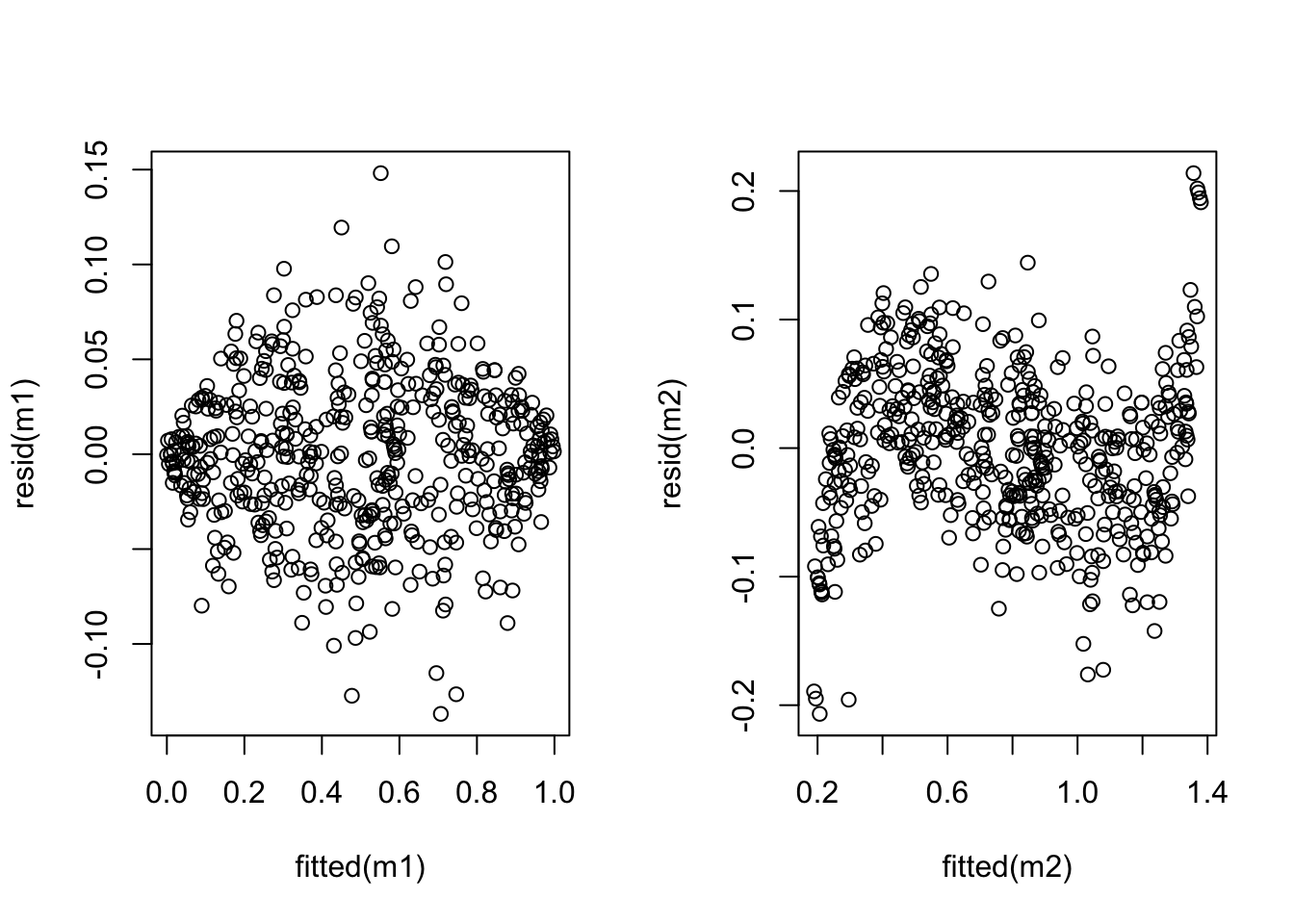The plot shows that the variance has indeed improved, while linearity has suffered (an S-shaped curve is now visible). Neither model is perfect and whether the transformation is beneficial or not depends on the particular circumstances.

Example 11.3 An electric utility company is interested in developing a model relating the peak hour demand ($$y$$, measured in kW) to total energy usage during the month ($$x$$, in kWh). A scatter plot of the data is shown below:

# data at https://www1.maths.leeds.ac.uk/~voss/2022/MATH3714/electric.dat
plot(d$V1, d$V2,
xlab="energy usage [kWh]", ylab="energy demand [kW]")
m1 <- lm(V2 ~ ., data = d)
abline(m1)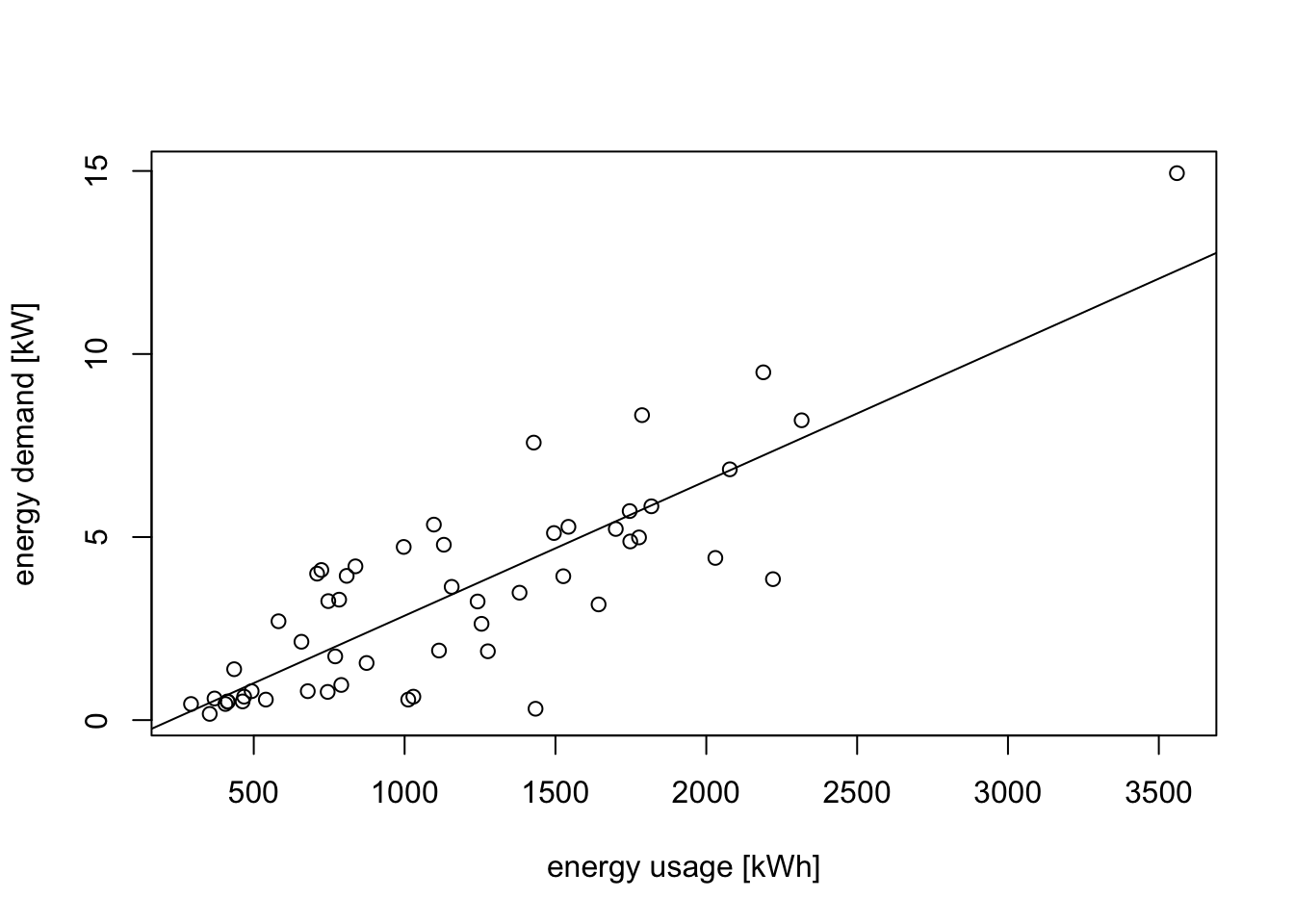A linear relationship looks plausible, but we can see that the spread of points around the regression line widens as energy usage increases. this is more clearly visible in a residual plot:

plot(fitted(m1), resid(m1),
xlab = "fitted values", ylab = "residuals")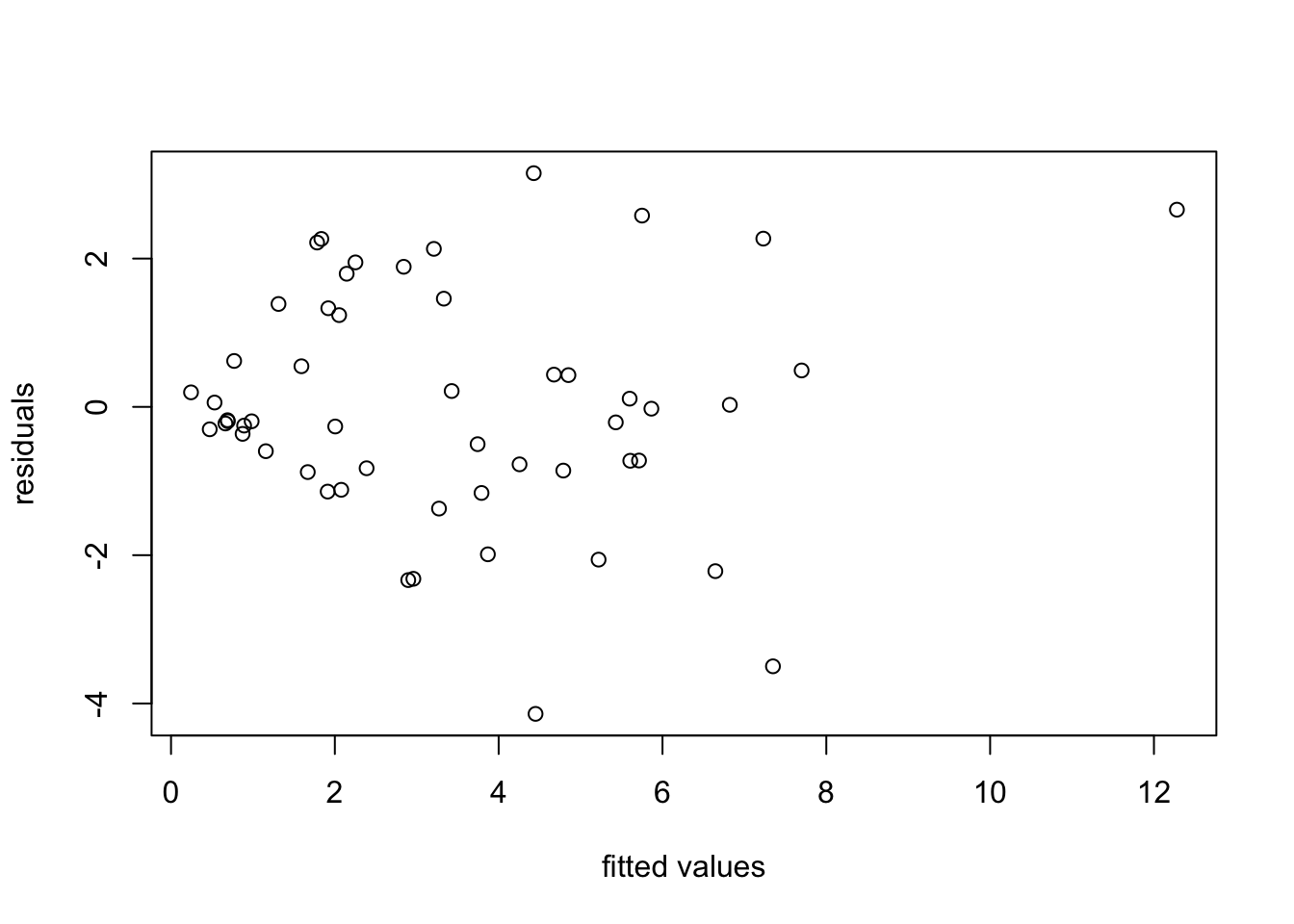We try a transformation of the data to stabilise the variance. From the “wedge” shape on the left hand side of the plot, it is clear that the variance $$\sigma^2$$ increases as $$y$$ increases. Thus we can try transformations like $$y' = \log(y)$$ or $$y' = \sqrt{y}$$. Taking the logarithm for illustration, we get

m2 <- lm(log(V2) ~ ., data = d)
plot(d$V1, log(d$V2),
xlab="energy usage [kWh]", ylab="log-transformed y")
abline(m2)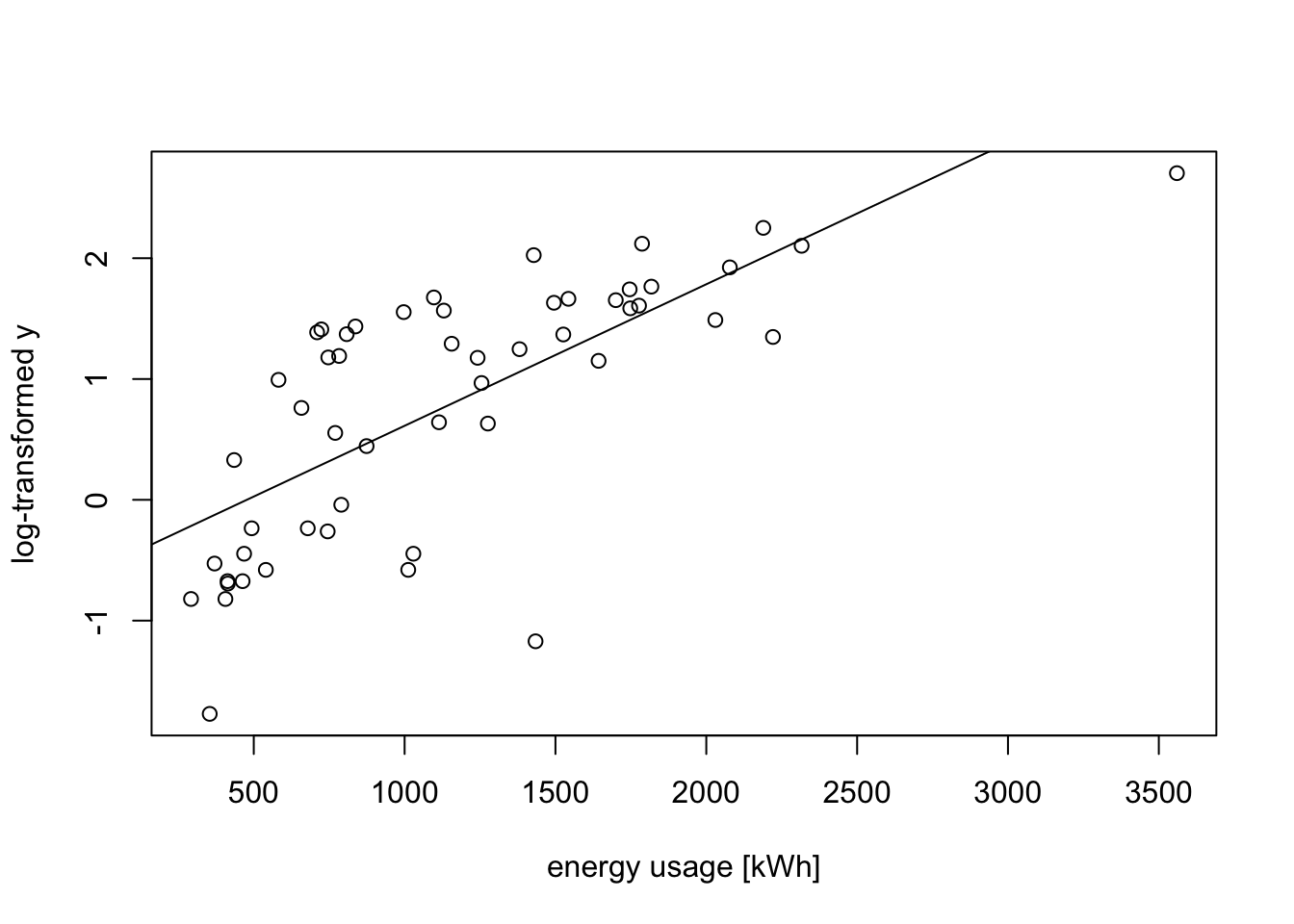plot(fitted(m2), resid(m2),
xlab = "fitted values", ylab = "residuals for log-transform")
abline(h = 0)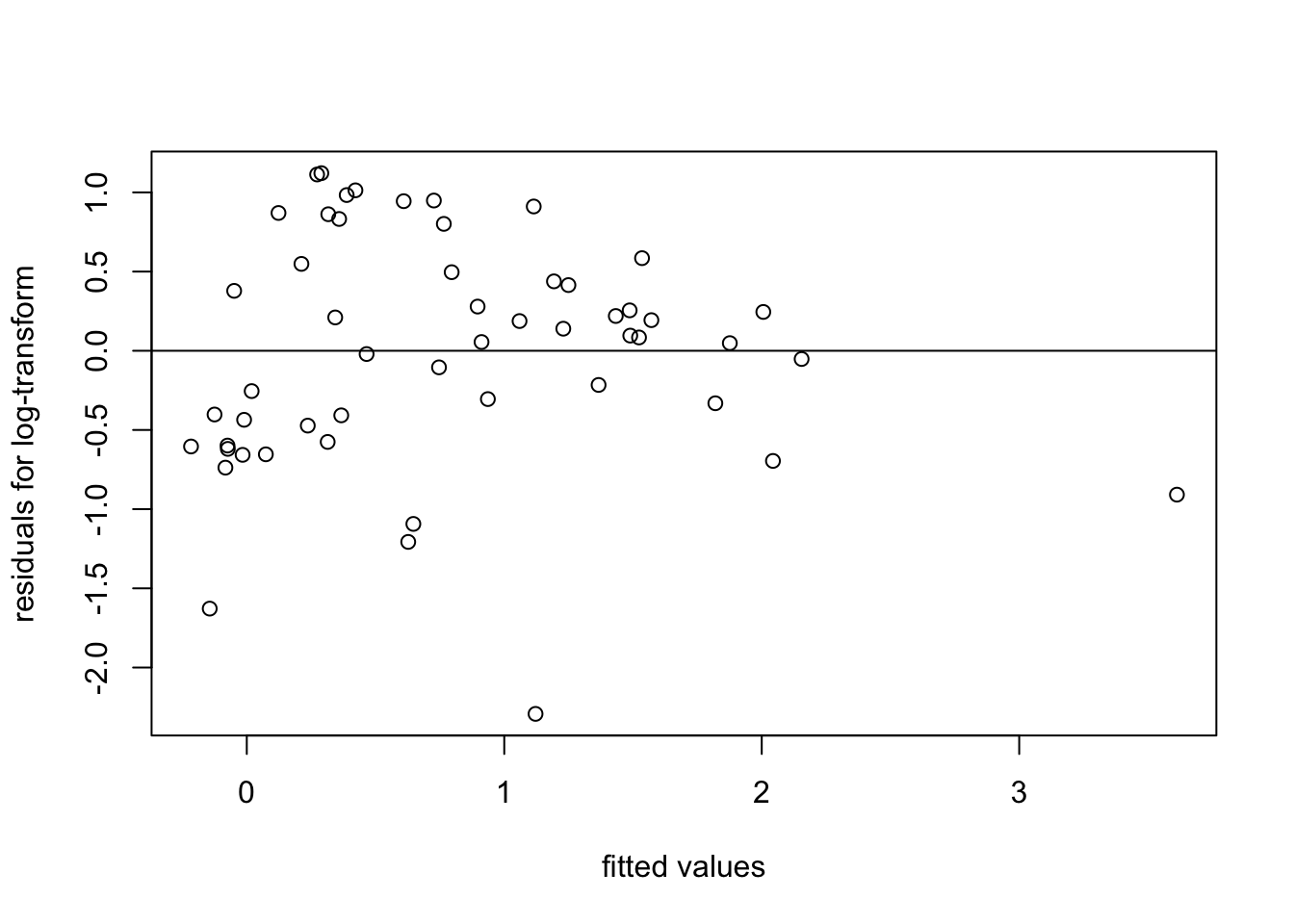The spread of residuals now looks somewhat more reasonable.

## 11.3 The Power Transform

A family of transformations for the response variable $$y$$ is given by the power transform. These transformations only apply to strictly positive data, i.e. $$y > 0$$, and are defined by $\begin{equation*} y^{(\lambda)} = \begin{cases} \fracy^\lambda-1}{\displaystyle\lambda g^{\lambda-1}} & \mbox{if \lambda\ne 0} \\ g\log y & \mbox{if \lambda=0} \end{cases} \end{equation*$ where $$g = (\prod_{i=1}^n y_i)^{1/n}$$ is the geometric mean.

The geometric mean is a constant (it does not depend on $$i$$) and is not needed for the power transform, but it is usually included to make values for different $$\lambda$$ more comparable. For $$\lambda = 1$$ the transform is $$y' = y - 1$$. This is a simple shift which has no effect on the fitted model, it just decreases the intercept by $$1$$ and leaves the residuals unchanged. Thus, this case is that same as applying no transformation.

Using Taylor approximation on the numerator in the definition of $$y^{(\lambda)}$$ we get $\begin{equation*} y^\lambda = \exp\bigl( \log(y^\lambda) \bigr) = \exp\bigl( \lambda \log(y) \bigr) \approx 1 + \lambda\log(y) + O\Bigl(\bigl(\lambda\log(y)\bigr)^2\Bigr), \end{equation*}$ where the $$O(\cdots)$$ stands for terms which are negligible as $$\lambda$$ converges to $$0$$. Thus the formula given for $$\lambda \neq 0$$ converges to the case for $$\lambda = 0$$ as $$\lambda$$ converges to $$0$$. This makes the transformation continuous as a function of $$\lambda$$.

A heuristic rule to find a range of $$\lambda$$ for which the power transform is appropriate is based on how the the residual sum of squares changes with $$\lambda$$: Let $\begin{equation*} r(\lambda) = \sum_{i=1}^n \bigl( y^{(\lambda)}_i - \hat y^{(\lambda)}_i \bigr)^2, \end{equation*}$ where $$\hat y^{(\lambda)}_i$$ denotes the fitted value for the model using the transformed data $$y^{(\lambda)}_i$$. It is easy to plot this function numerically. We want to choose $$\lambda$$ close to where the function has its minimum. The heuristic rule is to consider all values of $$\lambda$$ with $\begin{equation} r(\lambda) \leq r(\lambda_\mathrm{min}) \Bigl( 1 + \frac{t_{n-p-1}(\alpha/2)^2}{n-p-1} \Bigr), \tag{11.1} \end{equation}$ where $$\lambda_\mathrm{min}$$ is the value of $$\lambda$$ where the residual sum of squares has its minimum and $$t_{n-p-1}(\alpha/2)$$ is the $$(1-\alpha/2)$$-quantile of the $$t(n-p-1)$$-distribution. One can show that this is an approximate $$(1-\alpha)$$ confidence interval for $$\lambda$$. If we want to interpret the model, it is usually better to select a “simple” $$\lambda$$, e.g. $$\lambda=0.5$$ rather than using the “optimal” value $$\lambda_\mathrm{min}$$.

Example 11.4 Continuing from example 11.3 above, we can try a power transform for the data. We start by plotting $$r(\lambda)$$ as a function of $$\lambda$$, together with the cutoff suggested by equation (11.1):

x <- d$V1 y <- d$V2
gm <- exp(mean(log(y))) # more stable way to compute geometric mean
lambda <- seq(0.1, 1, length.out = 101)
for (i in seq_along(lambda)) {
li <- lambda[i]
y.prime <- (y^li - 1) / (li * gm^(li-1))
mi <- lm(y.prime ~ x)
}

n <- nrow(d) # 53
p <- 1
cutoff <- min(rss) * (1 + qt(0.975, n - p - 1)^2 / (n - p - 1))
abline(h = cutoff)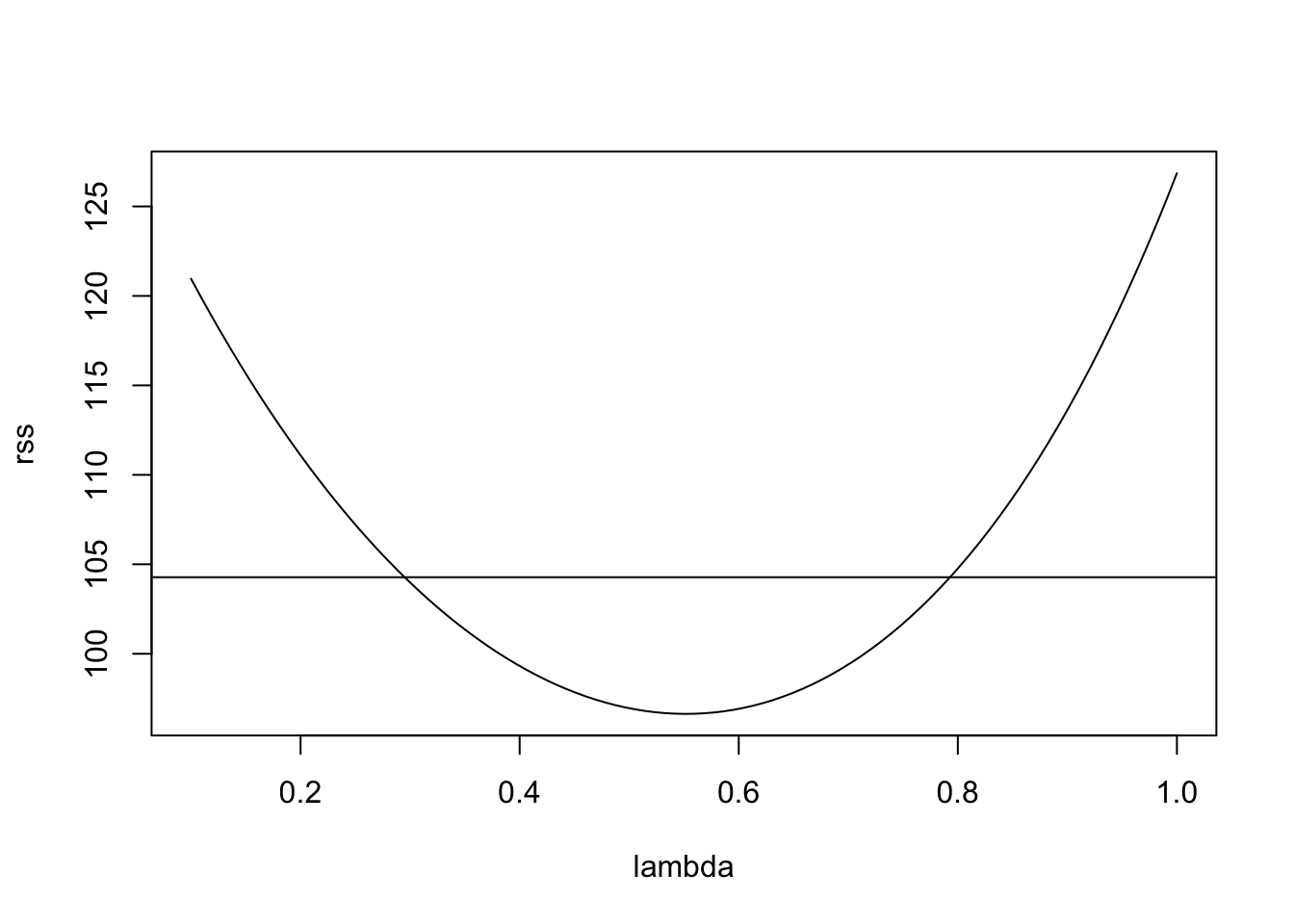This suggests the range of reasonable $$\lambda$$ values to be

range(lambda[which(rss <= cutoff)])
 0.298 0.784

The value $$\lambda = 1$$ (no transformation) is not contained in the interval, suggesting that a transformation may be helpful. Choosing a “simple” value inside the interval and close to the minimum, we try $$\lambda = 0.5$$. This leads to the following transformation: $\begin{equation*} y' = 2\sqrt{g} (\sqrt{y}-1). \end{equation*}$ Up to the shift and scaling, this just takes the square root of the data.

y.prime <- sqrt(y)
plot(x, y.prime,
xlab="energy usage [kWh]", ylab="sqrt-transformed y")
m3 <- lm(y.prime ~ x)
abline(m3)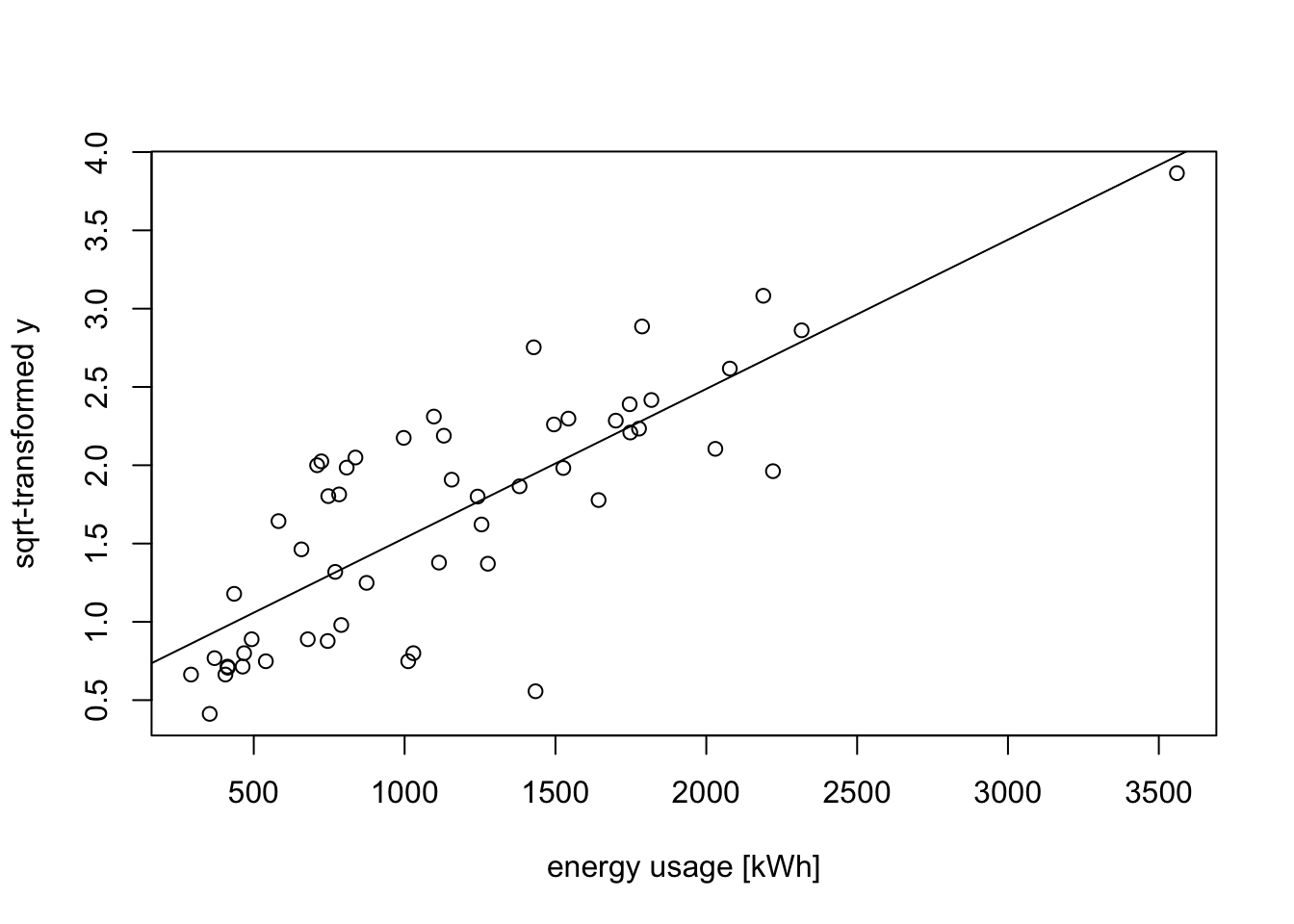To see whether the variance of the residuals has improved, we consider a residual plot:

plot(fitted(m3), resid(m3),
xlab = "fitted values", ylab = "residuals for sqrt-transform")
abline(h = 0)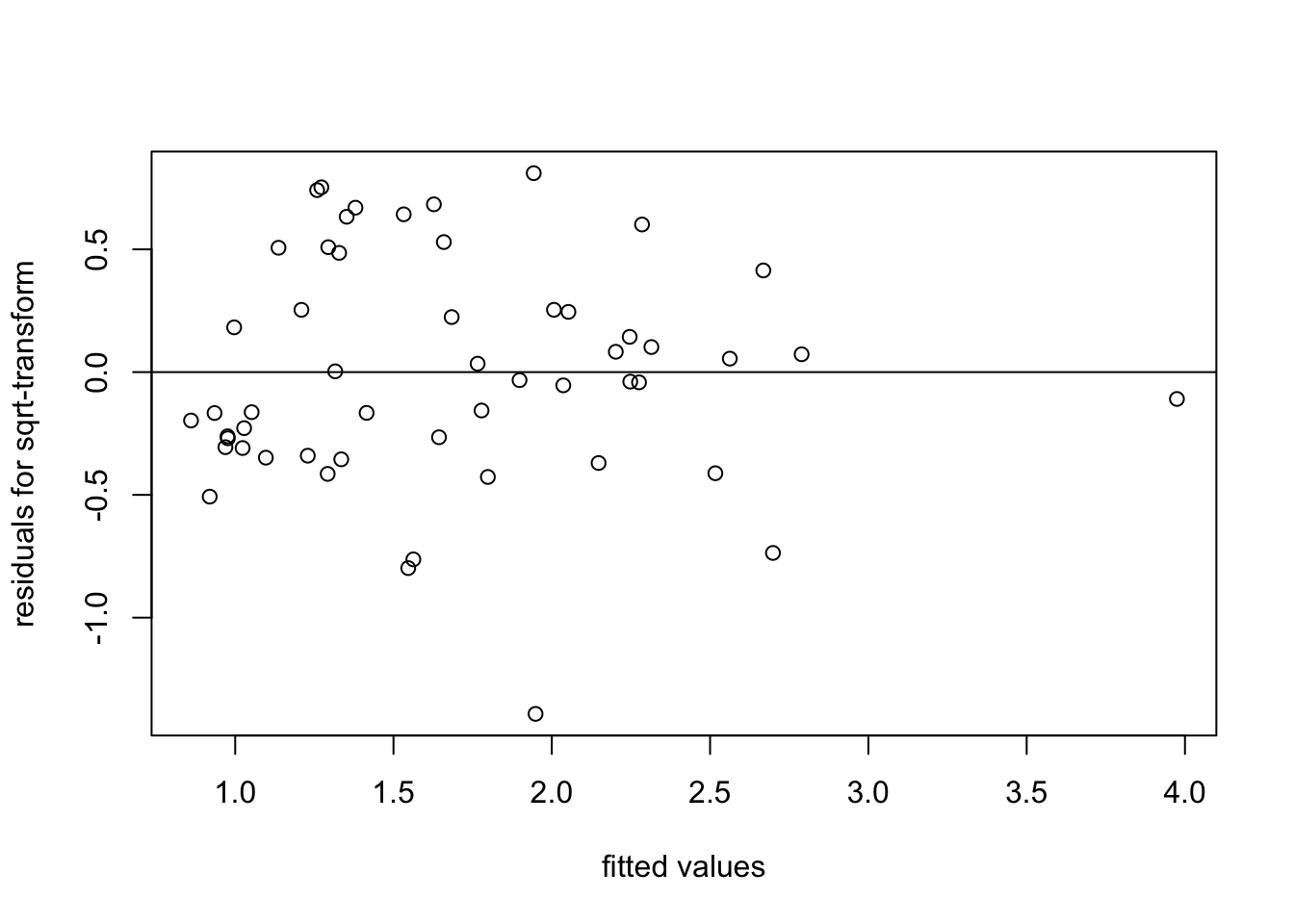We see that the spread of the residuals has indeed improved, when compared to fitting the original data.

## 11.4 Orthogonal Inputs

So far, this chapter has been concerned with what to do when there are problems with a given model. In some cases we have the chance to “plan ahead” so that problems are less likely to occur.

Collinearity occurs when there is high correlation amongst the explanatory variables. In some situations we have the opportunity to choose the design space $$X$$. In these cases, there are advantages in creating design variables which are orthogonal, thus avoiding or reducing problems caused by collinearity.

Example 11.5 Suppose we have observations $$(x_i, y_i)$$ for $$i\in \{1, \ldots, n\}$$ and we want to fit a polynomial model of the form $\begin{equation*} Y_i = \beta_0 + \beta_1 x_i + \beta_2 x_i^2 + \cdots + \beta_p x_i^p + \varepsilon_i. \end{equation*}$

If we use the actual inputs $$x$$ to create new variables, say $$x_j = x^j$$, then severe multicollinearity can arise. For example, for $\begin{equation*} x = (10000, 10001, \ldots, 10010), \end{equation*}$ we have $\begin{equation*} x^2 = 10^8 + (0, 20001, 40004, \ldots, 200100) \end{equation*}$ and $$\mathop{\mathrm{cor}}(x, x^2) \approx 1 - 9.74\times 10^{-9}$$:

x <- seq(10000, 10010)
cor(x, x^2)
 1
1 - cor(x, x^2)
 9.740257e-09
X <- cbind(1, x, x^2)
kappa(t(X) %*% X, exact = TRUE)
 1.47006e+23

The first result, for the correlation, is shown as $$1$$ due to rounding. The internal number representation of the correlation is actually less than $$1$$ and we can print the difference 1 - cor(x, x^2) to get the result stated above. Since this difference is close to $$0$$ (instead of close to $$1$$), R can now use scientific notation to show the very small result. The final line of the output shows that the condition number is greater than $$10^{23}$$, so the problem indeed suffers severe multicollinearity.

We can greatly improve the conditioning of the problem by using the centred data $$x^\ast_i = x_i - \bar x$$ before taking powers:

x.star <- x - mean(x)
cor(x.star, x.star^2)
 0
X.star <- cbind(1, x.star, x.star^2)
kappa(t(X.star) %*% X.star, exact = TRUE)
 408.7796

Now the correlation between $$x^\ast$$ and $$(x^\ast)^2$$ is exactly zero, and the condition number is much improved.

A more systematic approach to choose input variables for polynomial regression is to use orthogonal polynomials of the form \begin{align*} \psi_0(x) &= 1 \\ \psi_1(x) &= \alpha_{1,1} x + \alpha_{1,0} \\ \psi_2(x) &= \alpha_{2,2} x^2 + \alpha_{2,1} x + \alpha_{2,0} \\ &\vdots \\ \end{align*} where the coefficients $$\alpha_{j,k}$$ are chosen such that $\begin{equation*} \sum_{i=1}^n \psi_j(x_i) \psi_k(x_i) = 0 \end{equation*}$ for all $$j, k \in \{0, \ldots, p\}$$ with $$j \neq k$$. We can then use input variables $\begin{equation*} x_j = \psi_j(x) \end{equation*}$ for $$j \in \{0, \ldots, p\}$$ to get a design matrix $$X$$ such that \begin{align*} (X^\top X)_{jk} &= \sum_{i=1}^n X_{ij} X_{ik} \\ &= \sum_{i=1}^n \psi_j(x_i) \psi_k(x_i) \\ &= 0 \end{align*} for all $$j, k \in \{0, \ldots, p\}$$ with $$j \neq k$$. In this case the columns of $$X$$ are orthogonal and there is no multicollinearity. Our model is now \begin{align*} y_i &= \beta_0 + \beta_1 x_{i,1} + \cdots + \beta_p x_{i,p} + \varepsilon_i \\ &= \beta_0 \psi_0(x_i) + \beta_1 \psi_1(x_i) + \beta_2 \psi_2(x_i) + \cdots + \beta_p \psi_p(x_i) + \varepsilon_i. \end{align*} Since $$X^\top X$$ is diagonal, the inverse of this matrix is trivial to compute. If we denote the diagonal elements by $\begin{equation*} A_j = (X^\top X)_{jj}, \end{equation*}$ then $$X^\top X = \mathrm{diag}(A_0, \ldots, A_p)$$ and $$(X^\top X)^{-1} = \mathrm{diag}(1/A_0, \ldots, 1/A_p)$$. Thus we get \begin{align*} \hat\beta_j &= \bigl( (X^\top X)^{-1} X^\top y \bigr)_j \\ &= \frac{1}{A_j} \sum_{i=1}^n \psi_j(x_i) y_i \end{align*} and similarly, using equation (4.2), we find $\begin{equation*} \mathop{\mathrm{Var}}( \hat\beta_j ) = \frac{\sigma^2}{A_j}. \end{equation*}$

An additional advantage of this approach is, that we can add another term, $$\psi_{p+1}(x)$$, without changing the previous estimates of the regression coefficients. Since $$X^\top X$$ is diagonal, the change will not affect the estimates $$\hat\beta_j$$ for $$j\in \{0, \ldots, p\}$$ (but the change will affect $$\sigma^2$$).

Example 11.6 In R we can create specific orthogonal entries for polynomial regression using the command poly(x, p). The output of this function is a matrix with elements $$\psi_j(x_i)$$ for $$j \in \{1, \ldots, p\}$$ (omitting the intercept) and $$i \in \{1, \ldots, n\}$$. Continuing from the example above we find

x <- seq(10000, 10010)
X <- cbind(1, poly(x, 2)) # manually prepend a column of ones
round(t(X) %*% X, 5)
     1 2
11 0 0
1  0 1 0
2  0 0 1

Different from our previous approach, the last two columns are now also orthogonal to the columns of ones. This further improves the conditioning of the design matrix:

kappa(X, exact = TRUE)
 3.316625

We see that the use of orthogonal polynomials entirely eliminated any problems with multicollinearity.

Example 11.7 To illustrate the use of poly() for fitting a polynomial model to real data, we use the built-in cars dataset in R. This dataset contains historic data (from the 1920s) about the speed of cars (in mph) and the distances taken to stop (in ft). We first fit a second order polynomial to the data using the naive approach:

m1 <- lm(dist ~ speed + I(speed^2), data = cars)
plot(cars)
lines(predict(m1, newdata = data.frame(speed=seq(0, 30, l=31))),
col="blue")Checking the condition number, we see that there is severe multicollinearity:

kappa(m1, exact = TRUE)
 2160.976

We can also see that none of the coefficients is significantly different from zero, while the F-test rejects the hypothesis that the vector of all regression coefficients is zero. We have seen previously that this effect can be an indication of multicollinearity.

summary(m1)

Call:
lm(formula = dist ~ speed + I(speed^2), data = cars)

Residuals:
Min      1Q  Median      3Q     Max
-28.720  -9.184  -3.188   4.628  45.152

Coefficients:
Estimate Std. Error t value Pr(>|t|)
(Intercept)  2.47014   14.81716   0.167    0.868
speed        0.91329    2.03422   0.449    0.656
I(speed^2)   0.09996    0.06597   1.515    0.136

Residual standard error: 15.18 on 47 degrees of freedom
Multiple R-squared:  0.6673,    Adjusted R-squared:  0.6532
F-statistic: 47.14 on 2 and 47 DF,  p-value: 5.852e-12

We can fit an improved model using orthogonal polynomials. Since lm() automatically adds the intercept, we don’t need to prepend a column of ones to the poly() output here.

m2 <- lm(dist ~ poly(speed, 2), data = cars)
kappa(m2)
 6.670129

We see that in the new model there are no problems with multicollinearity any more. The new model also has coefficients which are significantly different from zero:

summary(m2)

Call:
lm(formula = dist ~ poly(speed, 2), data = cars)

Residuals:
Min      1Q  Median      3Q     Max
-28.720  -9.184  -3.188   4.628  45.152

Coefficients:
Estimate Std. Error t value Pr(>|t|)
(Intercept)       42.980      2.146  20.026  < 2e-16 ***
poly(speed, 2)1  145.552     15.176   9.591 1.21e-12 ***
poly(speed, 2)2   22.996     15.176   1.515    0.136
---
Signif. codes:  0 '***' 0.001 '**' 0.01 '*' 0.05 '.' 0.1 ' ' 1

Residual standard error: 15.18 on 47 degrees of freedom
Multiple R-squared:  0.6673,    Adjusted R-squared:  0.6532
F-statistic: 47.14 on 2 and 47 DF,  p-value: 5.852e-12

Summary

• We have seen different transformations which can be used to transform a nonlinear model into a linear one.
• We have discussed transformations which can stabilise the variance of the errors in the model.
• We have seen how orthogonal polynomials can be used to avoid multicollinearity in polynomial regression.